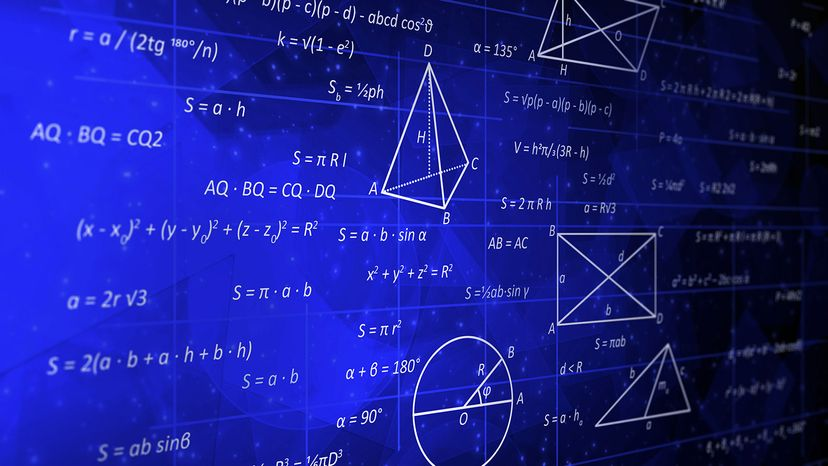# What Is the Area Formula for a Rectangle, a Triangle and a Circle?Area formulas give us the sizes of everything from baking pans to house plans. Knowing how to use them is key. GRADIENT BACKGROUND/Shutterstock

It's inevitable. At some point in every math class, someone asks the teacher (usually with an exasperated groan), "When are we going to use any of this math?" In the case of area formulas, the answer is: all the time. Knowing area formulas, as in the examples below, comes in handy in everyday life.

Learning to calculate the areas of different shapes — or any closed geometric figure — has nearly infinite real-world applications. Whether you're designing your own greeting cards, searching for a rectangular area rug that perfectly fits with the table in your room, building a treehouse, or painting a fence, knowing how to calculate area, or the space enclosed in any shape, is endlessly practical.

Contents

## What Is Area?

Area is the size of a flat shape's surface. It's the number of square units (inches, meters, miles) that would fit within a given space, whether that's a plot of land or the different shapes of a piece of construction paper.

Some of the most common shapes you'll need to measure for area are rectangles, triangles and circles. As you begin calculating areas, you may notice that you need some of the same components that perimeter formulas call for, such as length and width. So, what is the area formula for a rectangle, a triangle and a circle? Learn how to calculate the area for each using the area formulas in the examples below.

## How to Calculate the Area of a Rectangle

A rectangle comprises two sets of parallel sides. The formula for calculating the area within the four sides of a rectangle is one of the most useful equations you'll ever learn, and thankfully it's easy to remember: A = w × h. Find the area of this shape by multiplying width (w) by height (h). You'll also use this same formula to calculate the area of a square, which is essentially a rectangle in which all of the side lengths are the same.

### Example: Rectangle Area

The top of a rectangular coffee table with a width of 4 feet (1.21 meters) and a height of 3 feet (0.91 meters) would have an area of 12 square feet (1.11 square meters): A = 4 × 3 = 12.

## How to Calculate the Area of a TriangleTriangles are everywhere and knowing how to figure out a triangle's total area can come in handy. Dimaris/Shutterstock

The most common formula for triangle area, whether you're faced with the region enclosed in an isosceles triangle or an equilateral triangle, is calculated as: A = b × h / 2. Use this formula to find the area of a triangle by multiplying the length of the triangle's base (b) by its height (h), then dividing the product by 2. You can use any side of a triangle as its base.

There are other ways to calculate the area of the space inside a right triangle, or any triangle, depending on which measurements are given. If you know the lengths of two sides (a and b) and the angle between them (γ), you can use the area formula: A = a × b × sin(γ) / 2. If you know two angles (β and γ) and the side between them (a), apply the area formula: A = a² × sin(β) × sin(γ) / (2 × sin(β + γ)).

Lastly, if you know the lengths of all three sides (a, b and c), you can use the area formula: A = √( (a + b + c) × (-a + b + c) × (a - b + c) × (a + b - c) ) / 4.

### Example: Triangle Area

A triangle with a base of 3 inches (7.62 centimeters) and a height of 5 inches (12.7 centimeters) would have an area of 7.5 inches (19 centimeters): A = 3 × 5 / 2 = 7.5.

## How to Calculate the Area of a Circle

Use the following formula to calculate circle area: A = πr². Simply square the length of the radius (r), then multiply that number by pi (π).

Let's clarify a few things. A circle's radius is the distance of a straight line from the center point of the circle to a place on the circle's perimeter. Pi is a special number in mathematics that's typically rounded up to 3.14. (In actuality it's an irrational number with an infinite number of decimal places, but you don't have to worry about that.)